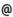## Babes-Bolyai University of Cluj-Napoca Faculty of Mathematics and Computer Science Study Cycle: Graduate SUBJECT

 Code Subject MMA0001 Mathematical Analysis 1
 Section Semester Hours: C+S+L Category Type Mathematics 1 3+2+0 fundamental compulsory Mathematics and Computer Science 1 3+2+0 fundamental compulsory Applied Mathematics 1 3+2+0 fundamental compulsory
 Teaching Staff in Charge
 Prof. DUCA Dorel, Ph.D.,  dducamath.ubbcluj.roLect. FINTA Zoltan, Ph.D.,  fzoltanmath.ubbcluj.roAssoc.Prof. DIACONU Adrian, Ph.D.,  adiaconumath.ubbcluj.ro
 Aims Getting to know the topology of the real axis and the differential and integral calculus of functions of one real variable.
 Content 1. Sets of numbers 1.1 Totally ordered commutative fields. 1.2 The set of real numbers. 1.3 The set of natural numbers. 1.4 The principle of the mathematical induction. 1.5 The set of integers. 1.6 The set of rational numbers. 1.7 The set of irrational numbers. 1.8 The symbols +∞ and -∞ . 1.9 Topology on real axes. 2. Sequences of real numbers 2.1 The limit of a sequence of real numbers, the uniqueness of the limit. 2.2 Passing to the limit in inequalities. 2.3 The convergence of the monotonous sequences. 2.4 Operations with sequences which have a limit. 2.5 Cauchy sequences. Cauchy@s theorem. 3. Series of real numbers 3.1 General notions. 3.2 Series with positive terms. 3.3 Series with random terms. 3.4 The convolute (Cauchy ) product of two series. 4. Limits of functions 4.1 The notion of the limit of a function at a point, the uniqueness of the limit. 4.2 Characterizations of the limit of a function. 4.3 Cauchy@s criterion of the existence of a limit of a function at a point. 4.4 Operations with functions which have a limit. 4.5 Passing to the limit in inequalities. 4.6 Side limits. 5. Continuous functions 5.1 The definition of continuity. 5.2 Characterization of the continuity of a function at a point. 5.3 Operations with functions continuous at a point. 5.4 Continuous functions on a set. 5.5 Continuous functions on a compact set. 5.6 Functions with Darboux@s property. 5.7 The continuity of the inverse function. 5.8 Uniformly continuous functions. 6. Derivable functions 6.1 The definition of the derivative function and of derivability. 6.2 The connection between derivability and continuity. 6.3 Side derivatives. 6.4 The geometric interpretation of the derivative. 6.5 The derivatives of some elementary functions. 6.6 Operations with derivable functions. 6.7 The derivability of the composed and of the converse functions. 6.8 Optimum points and local optimum points. Fermat@s theorem. 6.9 Darboux@s theorem. 6.10 Rolle@s theorem. 6.11 Cauchy@s theorem. 6.12 Mean value theorems. 6.13 L@Hôpital@s theorems. 6.14 Monotonous functions. The characterization of the monotony using derivatives. 6.15 High order derivatives 6.16 Convex functions. 6.17 Taylor@s formula. 6.18 Characterizations of optimum points using higher order derivatives. 7. Riemann integrable functions 7.1 Divisions of a compact interval. 7.2 The Riemann integral. 7.3 Characterizations of Riemann integrability using sequences of divisions. Cauchy@s criterion. 7.4 Darboux@s criterion on Riemann integrability. 7.5 Classes of Riemann integrable functions. 7.6 Lebesque@s criterion on Riemann integrability. 7.7 Operations with Riemann integrable functions. 7.8 Monotony properties of the Riemann integrable function. 7.9 Mean value theorems 7.10 The additivity of the integral function on the interval. 7.11 Primitives, the primitivability of continuous functions, the Leibniz-Newton formula. 7.12 Computing methods for the primitives. 7.13 The integrability of rational functions. 7.14 Methods of integration. 8. Sequences and series of functions 8.1 Point convergence and uniform convergence of a sequence of real functions. 8.2 Criteria on uniform convergences for sequences of functions. 8.3 The properties of the limit of a sequence of functions. 8.4 Point convergence and uniform convergence of a series of functions. 8.5 Criterions on uniform convergences for series of functions. 8.6 The property of the sum of a series of functions. 8.7 Power series.
 References 1. D. ANDRICA, D.I. DUCA, I. PURDEA, I. POP: Matematica de baza, Editura Studium, Cluj-Napoca, 2002 2. M. BALAZS, J. KOLUMBAN: Matematikai analizis, Dacia Konyvkiado, Kolozsvar-Napoca, 1978 3. W.W. BRECKNER: Analiză matematică. Topologia spaţiului Rn, Universitatea din Cluj-Napoca, Cluj-Napoca, 1985 4. S. COBZAS: Analiza matematica (Calcul diferential), Presa Universitara Clujeana, Cluj-Napoca, 1997 5. D.I. DUCA, E. DUCA: Analiza matematica. Culegere de probleme, Editura GIL, Zalau, 1999 6. L. LUPSA, L. BLAGA: Analiza matematica. Note de curs 1, Presa Universitara Clujeana, Editura Mega, Cluj-Napoca, 2003 7. H. LUENBURG: Vorlesungen uber Analysis, Manheim, Bibliographisches Institut, 1981 8. M. MEGAN: Bazele Analizei matematice, vol. 1,2,3, Editura Eurobit, 1997, 1997, 1998 9. GH. SIRETCHI: Calcul diferential si integral, vol. I si II, Editura Stiintifica si Enciclopedica, Bucuresti,1985 10. V.A. Zorich: Mathematical Analysis, Springer, Berlin, 2004
 Assessment The activity during the seminars and a test during the semester (20% of the final grade), and a written and oral exam at the end of the semester (80% of the final grade).
 Links: Syllabus for all subjects Romanian version for this subject Rtf format for this subject